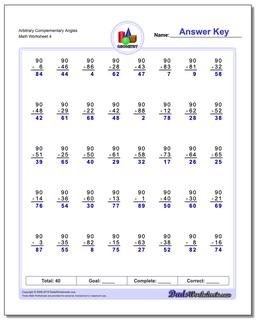# Math Worksheets: Basic Geometry: Basic Geometry: Arbitrary Complementary Angles (Fourth Worksheet)## Arbitrary Complementary Angles (Fourth Worksheet)

PropertyValue
DescriptionArbitrary Complementary Angles: Geometry worksheets with subtraction problems for finding complementary angles (Angles whose sum is 90 degrees). (Fourth Worksheet)
Resource TypeWorksheet